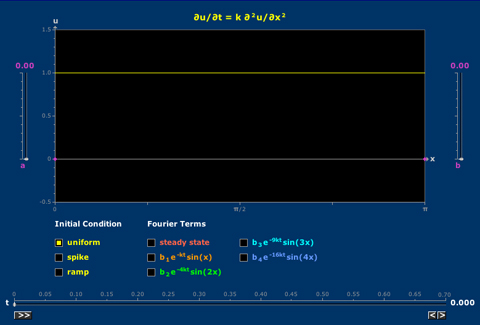# Heat Equation

The evolution of the temperature distribution on an insulated bar can be understood in terms of the Fourier decomposition of the initial condition.The evolution of the temperature distribution on an insulated bar can be understood in terms of the Fourier decomposition of the initial condition.

One Response to “Heat Equation”

1. Emmanuel Risler on June 9th, 2021 @ 12:19 pm

Thank you for this beautiful applet, very useful for many purposes (simplest example of a linear system with infinitely many eigenmodes, boundary conditions, introduction to Fourier series…).

Just a tiny remark concerning the notation: the letter k is often used to denote wavenumbers, therefore it might be relevant to use another notation for the diffusion coefficient in the applet (or to completely remove the diffusion coefficient). This way the letter k would be set free to denote wavenumbers in activities based on this applet.